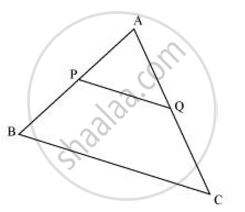Share

# Using Converse of Basic Proportionality Theorem, Prove that the Line Joining the Mid-points of Any Two Sides of a Triangle is Parallel to the Third Side. - CBSE Class 10 - Mathematics

#### Question

Using Converse of basic proportionality theorem, prove that the line joining the mid-points of any two sides of a triangle is parallel to the third side. (Recall that you have done it in Class IX).

#### SolutionConsider the given figure in which PQ is a line segment joining the mid-points P and Q of line AB and AC respectively.

i.e., AP = PB and AQ = QC

It can be observed that

(AP)/(PB) = 1/1

and (AQ)/(QC) = 1/1

∴ (AP)/(PB) = (AQ)/(QC)

Hence, by using basic proportionality theorem, we obtain

PQ || BC

Is there an error in this question or solution?

#### APPEARS IN

NCERT Solution for Mathematics Textbook for Class 10 (2019 to Current)
Chapter 6: Triangles
Ex. 6.20 | Q: 8 | Page no. 129

#### Video TutorialsVIEW ALL 

Solution Using Converse of Basic Proportionality Theorem, Prove that the Line Joining the Mid-points of Any Two Sides of a Triangle is Parallel to the Third Side. Concept: Similarity of Triangles.
S